Overall reaction to half reactions The overall unbalanced reactions are given below. Identify the oxidation and reduction half reactions and derive the balanced overall equation. Below is an example. In a particular battery cell the overall unbalanced reaction takes place.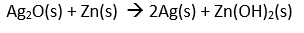Find the reduction and oxidation half equations and derive the balanced overall equation for the reaction.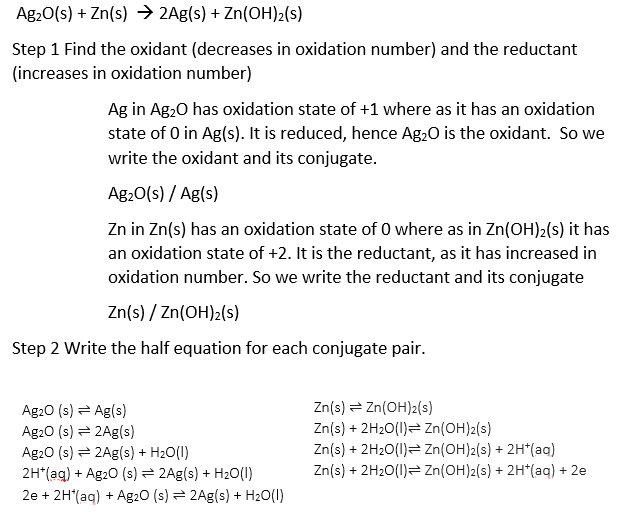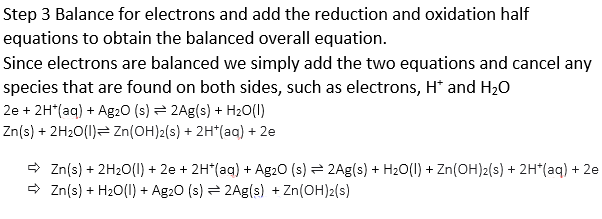Below are three overall unbalanced equations. Derive the balanced overall equation by finding the oxidation and reduction half equations first a)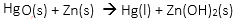Solution b)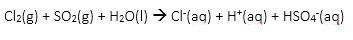Solution c)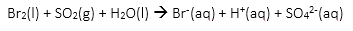Solution d)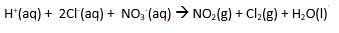SolutionFurther exercises/Solutions